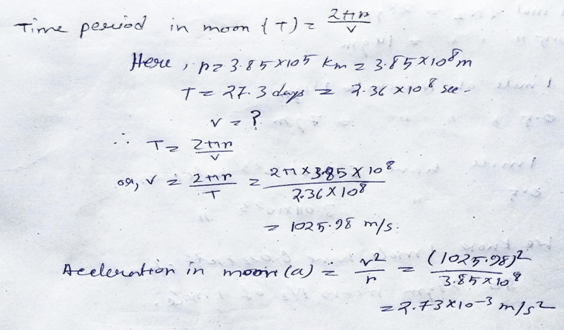# Telangana SCERT Class 9 Physical Science Chapter 5 Gravitation Solution

Telangana SCERT Class 9 Physical Science Chapter 5 Solution – Gravitation. Here in this post we provides Class 9 Physical Science Gravitation Telangana State Board Solution. Telangana State Board English Class IX Medium Students can download this Solution to Solve out Improve Your Learning Questions and Answers.

## Telangana State Board Class 9 Physical Science Chapter 5 Gravitation Solution:

### Gravitation

Exercise: –

Reflections on concepts

1) How do you explain that an object is in uniform circular motion (AS1)

Ans: –  As we all know that any object if running with the constant speed in a circular path then we will say that the object is moving with the uniform circular motion.

2) Calculate the acceleration of the moon towards earth’s centre. (AS1)

Ans: –3) Explain universal law of gravitation. (AS1)

Ans: – The universal law of gravitation state that the force is directly proportional to the masses and inversely proportional to the square of distance from this two. F=Gm1m2/r^2.

4) Explain some situations where the centre of gravity of man lies outside the body. (AS1)

Ans: – The centre of gravity lies outside the body if we doing some work bending like pulling some object from ground, bear some load with the back.

5) Explain why a long pole is more beneficial to the tight rope walker if the pole has slight bending. (AS7)

Ans: – The centre of gravity always plays an important role while we bearing some load. As a result of that when we use long pole for the tight rope walker then the centre of gravity changes and this is beneficial for us.

Application of Concepts –

1) A car moves with constant speed of 10 m/s in a circular path of radius 10m. The mass of the car is 1000 kg. How much is the required centripetal force for the car?

Ans: – The centripetal force (F) = mv^2 / r.

Here, m=1000kg, v= 10m/s, r=10cm.

So, F=1000× (10)^2/10 = 10^5N.

2) A ball is projected vertically up with a speed of 50 m/s. Find the maximum height, the time to reach the maximum height, and the speed at the maximum height(g=10 m/ s2) (AS1).

Ans: – As we all know, V= u + at;

Here, v= final velocity=0; u= initial velocity=50 m/s. a=g=10m/s^2.t=?.

So, 0= 50 – 10×t;

Or, t=5s.

And, v^2 = u^2 – 2as;

Or, 0= 50^2 – 2×10×s.

S= 125m.

3) Two spherical balls of mass 10 kg each are placed with their centres 10 cm apart. Find the gravitational force of attraction between them. (AS1)

Ans: – Gravitational force of attraction(F)= Gm1m2/ r^2.

Here, m1=m2=10kg, r=10cm=0.01m.

So, F= 6.67×10^11 × 10×10 / (0.01)^2

Or, F= 6.67×10^-7N.

4) A ball is dropped from a height. If it takes 0.2s to cross the last 6m before hitting the ground, find the height from which it is dropped. Take g = 10m/ s2. (AS1)

Ans: –5) What path will the moon take when the gravitational interaction between the moon and earth disappears? (AS2).

Ans: – The moon is connected or removing around the earth because of the centripetal force acting om them. As the moon and earth are connected by these gravitational forces it is moving otherwise the moon will gone away from root of the universe planet path.

6) Why is it easier to carry the same amount of water in two buckets, one in each hand rather than in a single bucket? (AS7)

Ans: – The centre of gravity works in the middle of the body of us while we are doing a work by carrying two same weight buckets in two hands. After doing this we felt light amount of weight as the centre of gravity is working through the body of us.

Higher Order Thinking Questions

1) A man is standing against a wall such that his right shoulder and right leg are in contact with the surface of the wall along his height. Can he raise his left leg at this position without moving his body away from the wall? Why? Explain. (AS7).

Ans: –  He couldn’t rise the left leg by this position of standing a at his right shoulder and right leg in contact with the surface of the wall along his height. As while doing this the centre of gravity will be outside the base of the body and then it will be difficult to stand in position.

2) An apple falls from a tree. An insect in the apple finds that the earth is falling towards it with an acceleration ‘g’. Who exerts the force needed to accelerate the earth with this acceleration? (AS7).

Ans: – The insect on apple while falling on the ground the it thought that the earth is coming toward it but in practically not possible so the apple while falling ground it exerts pressure on it of value g.

MULTIPLE CHOICE QUESTIONS.

1.) The acceleration which can change only the direction of velocity of a body is called

a) Acceleration due to gravity b) Uniform acceleration

c) Centripetal acceleration d) Deceleration.

Ans: – option (c).

2.)  The distance between the Earth and the Moon is

a) 3,84,400 Km b) 3,84,400 cm c) 84,000 Km d) 86,000 Km.

Ans: – option (a).

3.) The value of Universal Gravitational Constant is

a)6.67 x 10-11N.m2Kg-2 b) 9.8 m/ Sec 2

c) 6.67 x 10-12N.m2Kg-2 d) 981 m/ Sec2.

Ans: – option (a).

4.) The weight of an object whose mass is 1 Kg is

a) 1 Kg/m2 b) 9.8 m/Sec2 c) 9.8 N d) 9.8 N/m2.

Ans: – option (d).

Updated: January 25, 2022 — 4:23 pm